# 平稳性

ARIMA是自回归综合移动平均线的缩写。它是一类在时间序列数据中捕获一组不同标准时间结构的模型。

ARIMA模型是一类用于分析和预测时间序列数据的统计模型。它在使用上确实简化了，但是这个模型确实很强大。
ARIMA代表自回归综合移动平均。ARIMA模型的参数定义如下：
p：模型中包含的滞后观测数，也称为滞后阶数。
d：原始观测值的差异次数，也称为差分阶数。
q：移动平均线窗口的大小，也叫移动平均阶数。

# 步骤

1可视化时间序列数据
2确定日期是否平稳
3绘制相关图和自相关图
4根据数据建立ARIMA模型或季节ARIMA模型

``````df.head()

#更新表头
df.columns=["月份","销量"]

df.plot()``````# Python中的ARIMA模型、SARIMA模型和SARIMAX模型对时间序列预测

H0:零假设:这是一个关于总体的陈述，要么被认为是正确的，要么被用来提出一个论点。
H1:备选假设:与H0相矛盾，当我们拒绝H0时，我们得出的结论。

Ho:它是非平稳的

H1:它是平稳的

`` adfuller_test(df['销量'])``

``````ADF Test Statistic : -1.833
p-value : 0.363915
#Lags Used : 11
Number of Observations : 93``````

### 随时关注您喜欢的主题

``df['Sales First Difference'] = df['销量'] - df['销量'].shift(1) ``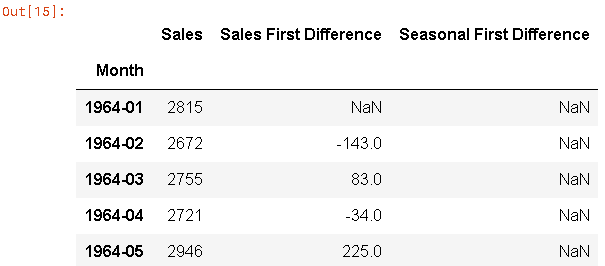``````# 再次测试数据是否平稳
``````ADF Test Statistic : -7.626619157213163
p-value : 2.060579696813685e-11
#Lags Used : 0
Number of Observations : 92``````

# 这里p值是2.06，表示拒绝零假设。所以数据是平稳的。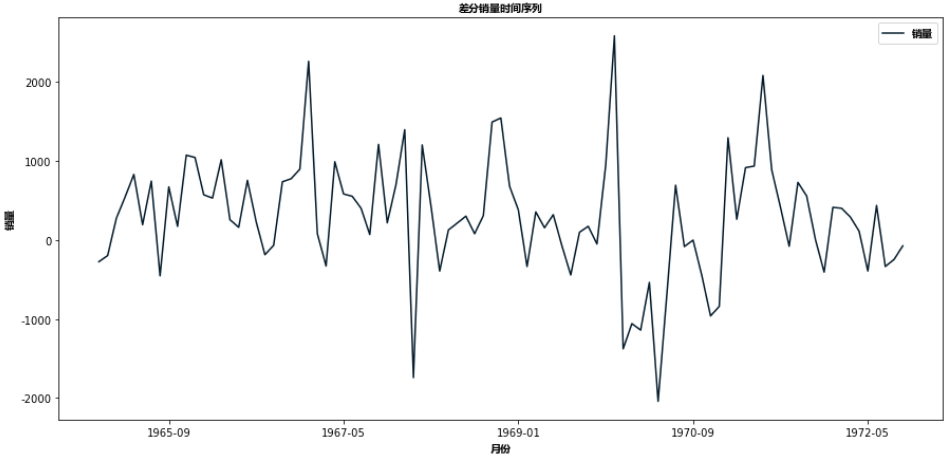# 自相关系数

``````autocorrelation_plot(df['销量'])
plt.show()``````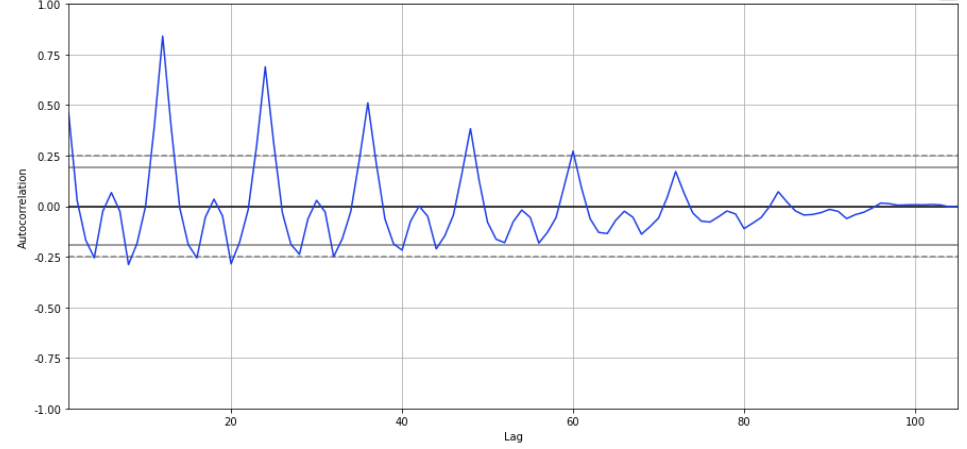``plot_acf(df['季节性一阶差分'].dropna(),lags=40,ax=ax1) ``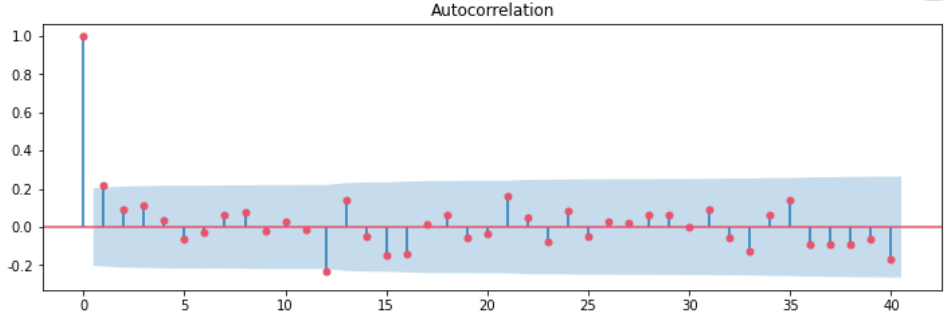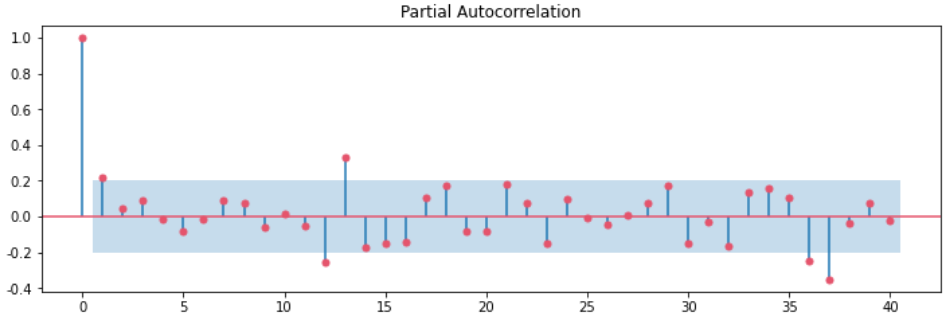# 建立ARIMA模型

``````#对于非季节性数据
#p=1, d=1, q=0 or 1

model=ARIMA(df['销量'],order=(1,1,1)) ``````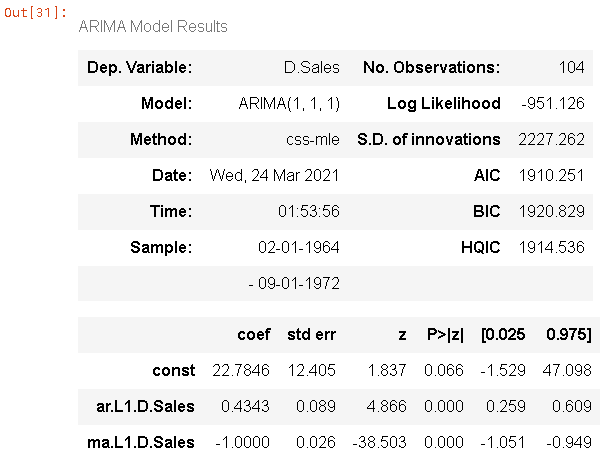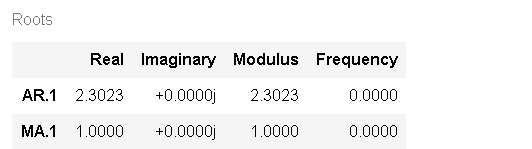``predict(start=90,end=103,dynamic=True) ``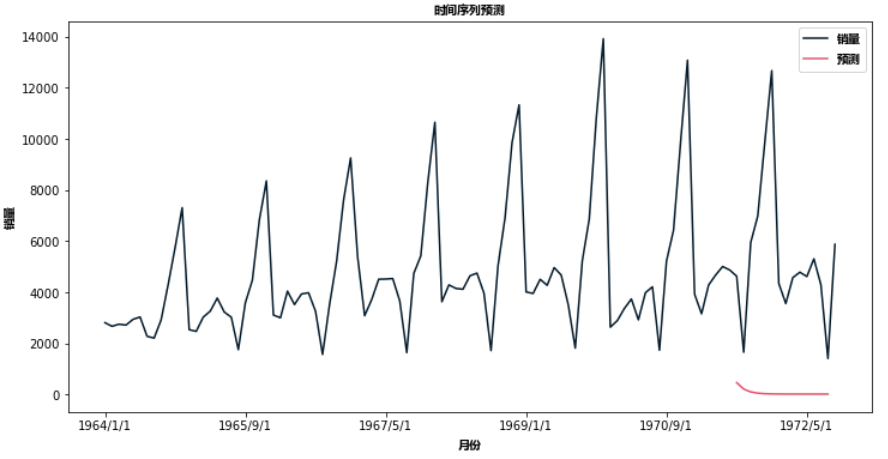`` plot(figsize=(12,8))``

# SARIMA模型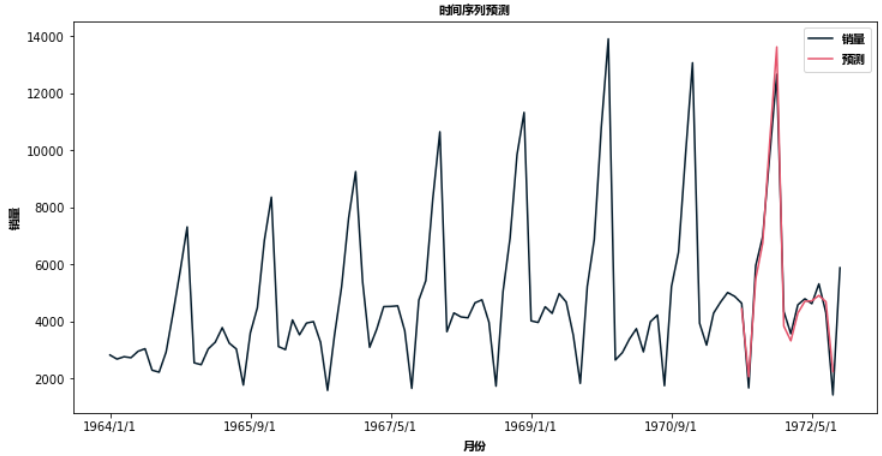`````` future_df['预测'] = results.predict(start = 104, end = 120, dynamic= True)
future_df.plot(figsize=(12, 8))``````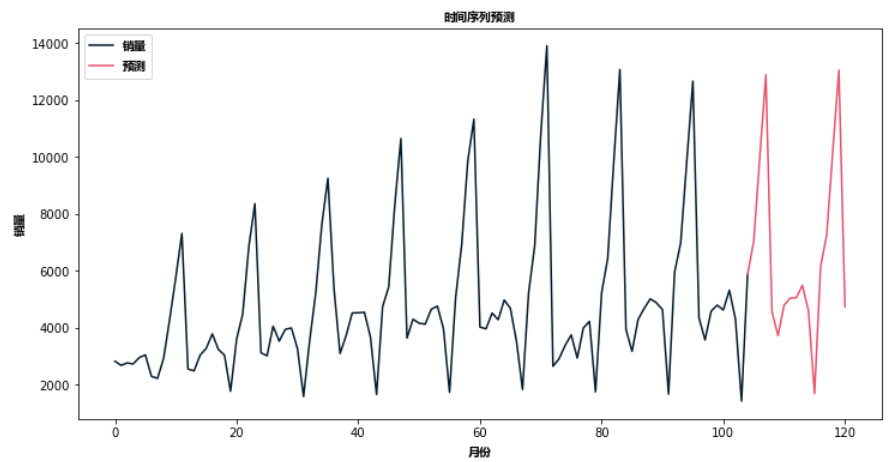# 结论

Kaizong Ye拓端研究室（TRL）的研究员。

​非常感谢您阅读本文，如需帮助请联系我们！QQ在线咨询

15121130882

0571-63341498

## 关注有关新文章的微信公众号

This will close in 0 seconds